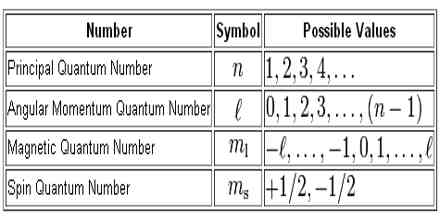Chemistry

# What are Quantum Numbers?The Quantum Numbers can be defined as the number by which size of energy level, shape of energy level, region of maximum probability of finding electrons and spin of electrons can be understood. There are four quantum numbers used to describe the exact position and nature of an electron. They are,

1. Principle Quantum Number (n)
2. Azimuthal (or Subsidiary) Quantum Number ()
3. Magnetic Quantum Number (m)
4. Spin Quantum Number (s)

These four quantum numbers are used to identify an electron completely of an atom. Because principal quantum number expresses the size of energy shell, subsidiary quantum number expresses the shape of the energy shell, magnetic quantum number expresses the three-dimensional orientation of the energy shell and spin quantum number expresses the spin of the electron.

The role of four quantum numbers to identify an electron of an atom is explained below.

1. Principal Quantum Number (n)

It expresses the principal energy level or the energy shells which represent the size of energy shell, it is denoted by n.

Where, n=1,2,3,4,5….etc.(Principal energy shell) They are also termed as K,L,M,N….etc shells.

2. Azimuthal (or Subsidiary) Quantum Numbers ()

(also known as the angular quantum number or orbital quantum number) Azimuthal quantum number express the angular momentum of the electron and it represents the shape of energy shell. It is denoted by .

Where,

ℓ=0 to (n-1) and n is the principal quantum number.

For example:

For n=1, ℓ= 0

For n=2, ℓ= 0, 1

For n=3, ℓ= 0, 1, 2 and so on.

Azimuthal Quantum number, ℓ=0 is denoted by S-orbital (s-sharp), ℓ= 1 is denoted by p-orbital (p-principal)

ℓ= 2 is denoted by d-orbital (d-diffuse)

ℓ= 3 is denoted by f-orbital (f-fundamental)

3. Magnetic quantum number (m)

This quantum number describes the behavior of the electron in magnetic fields. Under the influence of the external magnetic field, the electrons in a given subshell orient themselves in certain preferred regions of space around the nucleus. These are called orbitals. Thus this quantum number gives the number of orbitals in a given subshell. It is designed by m. The allowed values of m depend upon the value of ℓ. For a given value of ℓ, m can have values – ℓ through 0 to +ℓ.

i.e. m = – ℓ……0……+ℓ. In other words, there are (2ℓ + ℓ) values of m for each value of ℓ.

For example, if ℓ=0, m has only one value, i.e. m=0.

i.e. S – subshell has only one orbital called S-orbital.

If ℓ=1, m may be, -1, 0, +1

i.e. p – subshell contains three orbitals called p-orbital. These are indicated by numerical subscripts (P1…….P0…….P1) or these are designed as 2Px, 2py and 2Pz

If ℓ=2, m may be -2, -1, 0, +1, +2

i.e. d – subshell contains five orbitals called d-orbital.

4. Spin quantum number (s)

Spin Quantum Number express the spin of the electron on their own axis. One electron in an orbital spin clockwise and the other anti-clockwise.

They are denoted by S=-1/2 and +1/2